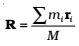The centre of mass of a system of particles moves as if all the mass of the system was concentrated at the centre of mass and all the external forces were applied at that point.

For rigid bodies, the centre of mass is independent of the state of the body i.e., whether it is in rest or in accelerated motion centre of mass will remain same.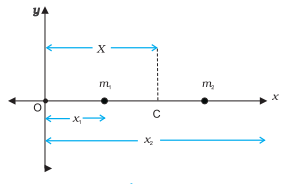For simplicity, we shall start with a two particle system. We shall take the line joining the two particles to be the x– axis.

Let the distances of the two particles be x1 and x2 respectively from some origin O. Let m1 and m2 be respectively the masses of the two particles. The centre of mass of the system is that point C which is at a distance X from O, where X is given by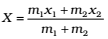————–(1)

In Eq. (1), X can be regarded as the mass weighted mean of x1and x2.

Case 1: If the two particles have the same mass m1 = m2 = m. then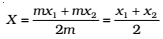Thus, for two particles of equal mass, the centre of mass lies exactly midway between them.

Case 2: If we have n particles of masses m1, m2, …mn respectively, along a straight line taken as the x– axis, then by definition the position of the centre of the mass of the system of particles is given by—————-(2)

where x1 , x2 ,…xn are the distances of the particles from the origin; X is also measured from the same origin. The symbol ∑ (the Greek letter sigma) denotes summation, in this case over n particles. The sum is the total mass of the system.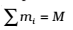Let ri be the position vector of the ith particle and R be the position vector of the centre of mass: